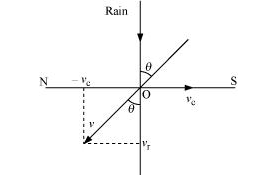# Rain is falling vertically with a speed ofQuestion.
Rain is falling vertically with a speed of $30 \mathrm{~m} \mathrm{~s}^{-1}$. A woman rides a bicycle with a speed of $10 \mathrm{~m} \mathrm{~s}^{-1}$ in the north to south direction. What is the direction in which she should hold her umbrella?

solution:

The described situation is shown in the given figure.Here,

$v_{c}=$ Velocity of the cyclist

$v_{\mathrm{r}}=$ Velocity of falling rain

In order to protect herself from the rain, the woman must hold her umbrella in the direction of the relative velocity (v) of the rain with respect to the woman.

$\vec{v}=\overrightarrow{v_{r}}+\left(-\overrightarrow{v_{c}}\right)$

Resultant, $|\mathrm{v}|=\sqrt{\mathrm{v}_{\mathrm{r}}{ }^{2}+\mathrm{v}_{\mathrm{c}}{ }^{2}}$

$|\mathrm{v}|=\sqrt{30^{2}+10^{2}}$

$|v|=\sqrt{900+100}$

$|\mathrm{v}|=\sqrt{1000}=10 \sqrt{10} \mathrm{~m}$

$\tan \theta=\frac{v_{c}}{v_{r}}=\frac{10}{30}$

$\theta=\tan ^{-1}\left(\frac{1}{3}\right)$

$\theta=\tan ^{-1}(0.333) \approx 18^{\circ}$

Hence, the woman must hold the umbrella toward the south, at an angle of nearly $18^{\circ}$ with the vertical.